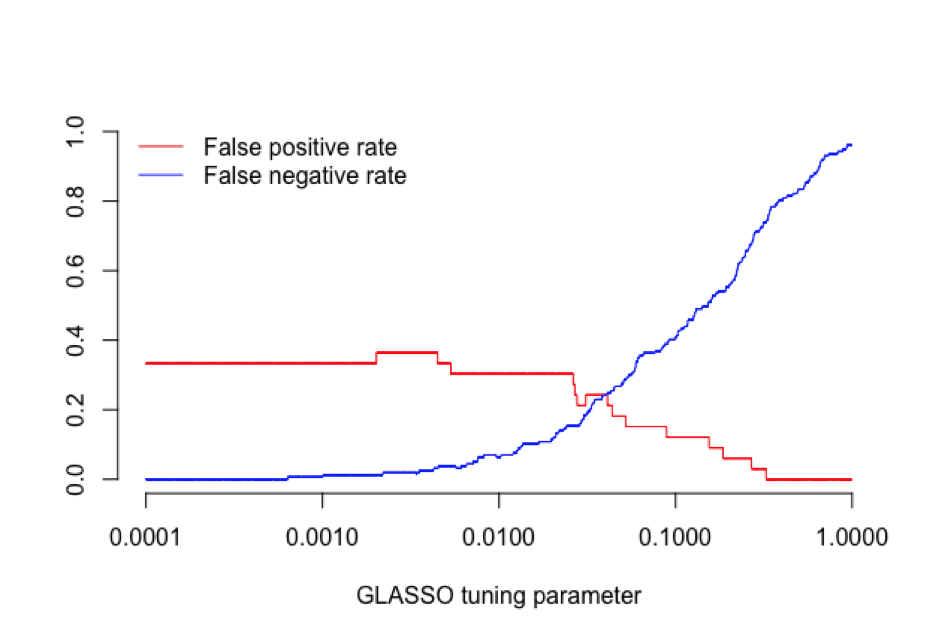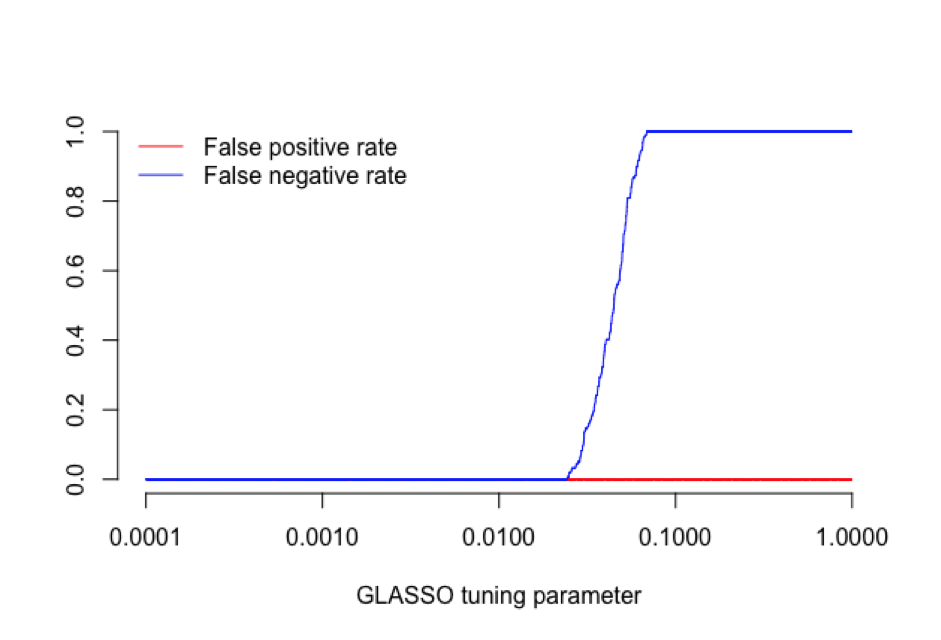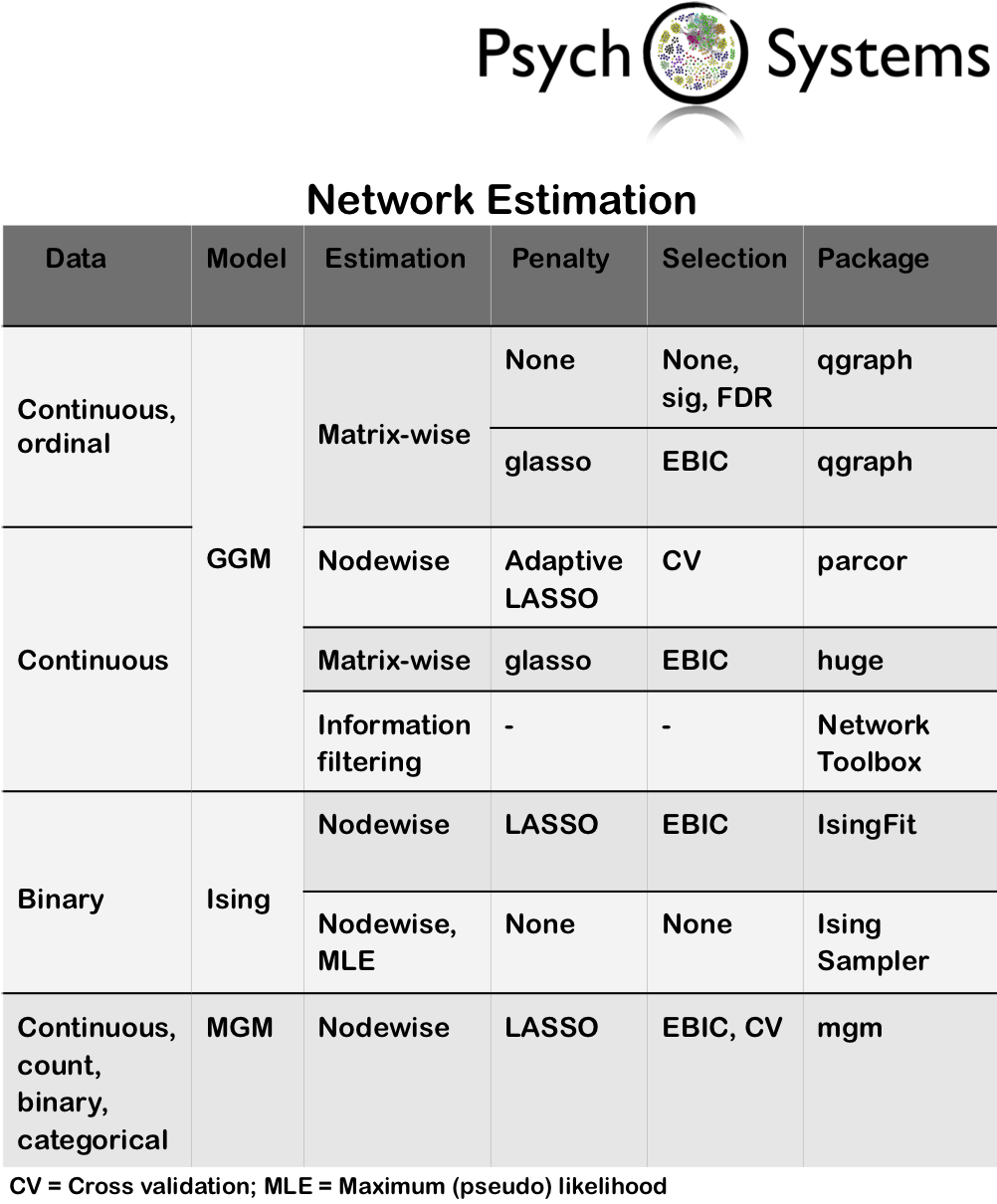# Recent developments on the performance of graphical LASSO networks

This post was written by Sacha Epskamp, Gaby Lunansky, PIa Tio and Denny Borsboom, on behalf of the Psychosystems lab group.

Currently, many network models are estimated using LASSO regularization. For ordinal and continuous variables, a popular option is to use the graphical LASSO (GLASSO), in which the network is estimated by estimating a sparse inverse of the variance-covariance matrix. For a description of this method, see our recently published tutorial paper on this topic. Last week we were informed of the new pre-print archive of Donald Williams and Philippe Rast, who show that under certain conditions the GLASSO, coupled with EBIC model selection (EBICglasso), can have lower specificity than expected. Specificity is defined as: 1 – the probability of estimating an edge that is not in the true model. Thus, the results of Williams and Rast indicate that in certain cases edges may be retrieved that are not representative of true edges. This is interesting, as LASSO methods usually show high specificity all-around.

We have investigated these claims and replicated the findings using the independent implementations of EBICglasso in both the qgraph and huge packages. This effect seems to only occur in network structures that are very dense (much denser than typical simulations and proofs regarding GLASSO investigate) and feature many very small edges, blurring the line between true and false effects. We already noted a noteworthy lower specificity than expected in our tutorial paper when discussing tools one may use to investigate these properties for a given network structure, and hypothesized that this may be an effect of a dense network structure with many small edges. The results of Williams and Rast seem to confirm this speculation by taking this effect to the extreme.

What is the problem and how can it be solved?

The main problem lies in that in some cases, the GLASSO algorithm may retrieve very small false edges, which are not present in the true network under which the data are simulated. There are two reasons why this may occur:

1. For certain tuning parameters, GLASSO identifies the correct model. However, the EBIC or cross-validation selects the wrong tuning parameter.
2. GLASSO is incapable of selecting the right model for any tuning parameter even with infinite sample size, and as a result any selection procedure is doomed to fail.

Case 1 is interesting, and may be the reason why regularized EBICglasso estimation has high specificity but does not always converge to the true model. This case may lead to a drop in specificity to about 0.6 – 0.7 and seems to occur in dense networks. We hypothesize that the added penalty biases parameters so much EBIC tends to select a slightly denser model to compensate. We are currently working out ways to improve tuning parameter selection, including running more simulations on preferred EBIC hyperparameters in denser networks than usually studied, theoretically derived thresholding rules, and non-regularized model selection procedures.

Case 2 seems to be the case in which Williams and Rast operate, which is harder to tackle. To exemplify this problem, we generated a 20% sparse graph using the codes kindly provided by Williams and Rast, and entered the true implied variance-covariance matrix into glasso. Inverting this true matrix and thresholding at an arbitrary small number returns the correct graph. However, at no tuning parameter the GLASSO algorithm is capable of retrieving this graph:The codes for this example are available online. It is striking that at no point GLASSO captures the true model, even though the true variance-covariance matrix (corresponding to N = infinite) was used as input. This is not common. Using the exact same codes, an 80% dense network parameterized as according to Tin & Li (2011), shows a strikingly different picture:showing that the true model is retrieved for some GLASSO tuning parameters. We are currently investigating whether we can identify cases in which GLASSO is incapable of retrieving the correct model at infinite sample size, and hypothesize this may be due to (a) very low partial correlations used in the generating structure, leading to a blurred line between true and false edges, and (b) relatively large partial correlations or implied marginal correlations, leading to inversion problems. We hope anyone may have feedback on why this set of networks fails in GLASSO estimation and how such troublesome cases may be discovered from empirical data.

Why can sparsity impact GLASSO performance?

Many network estimation techniques, including the GLASSO, rely on the assumption of sparsity, also termed the bet on sparsity. That is, these methods work very well assuming the true model is sparse (e.g. contains relatively few edges). However, when the true model is not sparse, LASSO may lead to unexpected results. Most simulation studies and mathematical proofs studied network retrieval in sparse settings. As more psychological network studies are published, however, one consistent finding seems to be that psychological networks are less sparse than expected. At high sample sizes, most studies identify many small edges near zero. This finding led to a change in the qgraph default for the lower bound on the sparsity of networks compared (lambda.min.ratio), which when set to 0.1 leads to networks being estimated that are too sparse. The default is now 0.01, which leads to better sensitivity but also a small decrease in specificity. Williams and Rast set this value to 0.001 in some cases, which should mark again an increase in sensitivity but perhaps also a decrease in specificity.

Are graphical LASSO networks unstable?

Fortunately, even in the extreme cases discussed by Williams and Rast, low specificity arises exclusively from the fact that very weak edges might be incorrectly included in the graph. In a qgraph plot, for instance, these would be nearly invisible. As a result, the general structure or substantive interpretation of the network is not affected. GLASSO results, like other statistical methods, are not intrinsically stable or unstable, and follow up stability and accuracy checks should always be performed after fitting a network. If the estimated network is stable and accurate, there is no reason to doubt the interpretation based on the work of Williams and Rast, except when the interpretation relied on thorough investigation of the weakest edges in the network (possibly made visible by removing the fading of edges in displaying them). Of note, the bootstrapping methods also allow for checking if an edge is significantly different from zero. We argue against this procedure, as it would lead to double thresholding a network and a severe loss of sensitivity. But if the goal is to retrieve small edges, it may be warranted to perform the check if zero is contained in the confidence interval of the regularized edge parameter.

Does this affect the replicability of networks?

Replicability and model selection are related but do not refer to the same methodological criterium. The recent findings regarding the GLASSO algorithm appeal to model quality: when simulating data from a ‘true model’, we can check whether results from an estimation method converge to this true model with increasing sample size (Borsboom et al., in press). Under certain circumstances, GLASSO algorithm retrieves very small edges which are not present in the true model under which the data are simulated (e.g. the edges are false-positives).  Replicability concerns finding the same results in different empirical samples. Naturally, if the GLASSO algorithm indeed retrieves small false-positive edges, this will affect replicability of these small edges over different empirical samples. However, since these edges shouldn’t be there in the first place, the origin of the issue does not concern replicability but model selection. While we investigate these results further, an important take-home message of the work of Williams and Rast is that researchers who aim to use network models to identify very small effects (when it is plausible to assume that the ‘true model’ might be dense with many small edges), may wish to consider other estimation methods such as node-wise estimation or non-regularized model selection discussed below.

Are all networks estimated using the graphical LASSO?

No. GLASSO is only one of many options that are available to estimate undirected network models from data. GLASSO is often used because it (a) is fast, (b) has high power in detecting edges, (c) selects the entire model in one step, and (d) only requires a covariance matrix as input, allowing to compute networks from polychoric correlations (ordinal data) and facilitating reproducibility of results. Outside the GLASSO, another option for regularized network estimation is to perform (logistic) regressions of every variable on all other variables, and combining the resulting regression parameters (proportional to edge weights) in the network. This is called nodewise estimation, and is at the core of several often used estimation methods such as the adaptive LASSO, IsingFit, and mixed graphical models (MGM). While nodewise regression estimation has less power than GLASSO, there are cases in which GLASSO fails but nodewise regressions do not (Ravikumar et al., 2008). Our preliminary simulations showed that nodewise estimation methods retain high specificity in the particular case identified by Williams and Rast where the specificity of GLASSO drops.  In addition to LASSO estimation methods that aim to retrieve sparse models, other regularization techniques exist that instead aim to estimate low-rank dense networks. Still another option is to use elastic-net estimation, which compromises between estimating sparse and dense networks.

Of note, and as Williams and Rast describe clearly, networks can also be estimated using non-regularized methods. Two main methods by which this can be done are (a) estimating a saturated network and subsequently removing edges that are below some threshold (e.g., based on significance tests, as argued by Williams and Rast), and (b) selecting between several estimated models in which edge-parameters are estimated without regularization. Both have already been discussed in the blog post that also introduced GLASSO estimation in the qgraph package. By using the ‘threshold’ argument in qgraph and bootnet one can threshold edges based on significance (possibly after correcting for multiple tests) or false discovery rates. A downside of thresholding, however, is that no model selection is performed, which leads to estimated edges that do not take into account other edges that are set to zero. A (rather slow) step-wise model selection procedure has been implemented in qgraph in the function ‘findGraph’. We are currently working on a faster non-regularized model selection procedure, in which the GLASSO algorithm is first used to generate a limited set of models (e.g., 100), which are subsequently refitted without regularization. Preliminary simulations show that this procedure is more conservative than regularized GLASSO and often converges to the true model with high sample sizes.

The bootnet package contains many default sets to facilitate computing networks using different estimation procedures in a unified framework. The table below provides an overview of methods currently implemented as default sets and when they can be used. If you would like us to implement more default sets, please let us know!References

Borsboom, D., Robinaugh, D. J.,  The Psychosystems Group, Rhemtulla M. & Cramer, A. O. J. (in press). Robustness and replicability of psychopathology networks. World Psychiatry.

Foygel, R., & Drton, M. (2010). Extended Bayesian information criteria for Gaussian graphical models. Advances in neural information processing systems, 604-612.

Ravikumar, P., Raskutti, G., Wainwright, M. J.,  & Yu, B. (2008). High-dimensional covariance estimation by minimizing l1-penalized log-determinant divergence. Electronic Journal of Statistics, 5:935–980.

Yin, J., & Li, H. (2011). A sparse conditional gaussian graphical model for analysis of genetical genomics data. The annals of applied statistics, 5(4), 2630-2650.